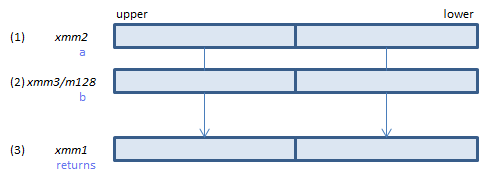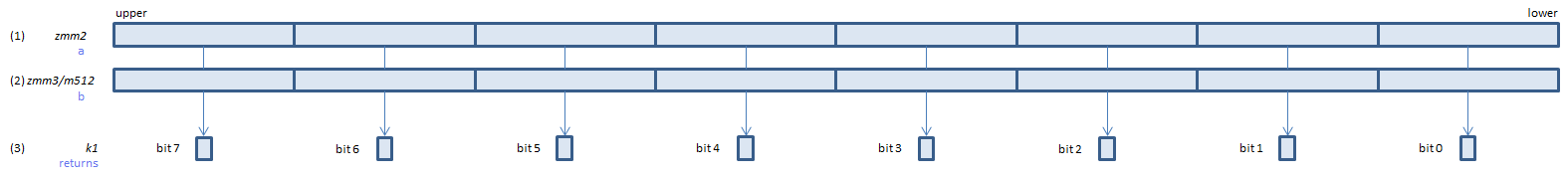﻿ vcmple_oqpd

## VCMPLE_OQPD - CoMPare Less Equal Ordered Quiet Packed Double

VCMPLE_OQPD xmm1, xmm2, xmm3/m128    (V1
__m128d _mm_cmp_pd(__m128d a, __m128d b, _CMP_LE_OQ)For each double, if (1) <= (2) set 1, else set 0, to all bits of the corresponding double of (3).
VCMPLE_OQPD ymm1, ymm2, ymm3/m256    (V1
__m256d _mm256_cmp_pd(__m256d a, __m256d b, _CMP_LE_OQ)For each double, if (1) <= (2) set 1, else set 0, to all bits of the corresponding double of (3).
VCMPLE_OQPD k1{k2}, xmm2, xmm3/m128/m64bcst    (V5+VLFor each double, if (1) <= (2) set 1, else set 0, to the corresponding bit of (3).
If k2 bit is 0, the comparison is not done and the corresponding bit of (3) is set to zero. Upper bits of (3) are zero cleared.
VCMPLE_OQPD k1{k2}, ymm2, ymm3/m256/m64bcst    (V5+VLFor each double, if (1) <= (2) set 1, else set 0, to the corresponding bit of (3).
If k2 bit is 0, the comparison is not done and the corresponding bit of (3) is set to zero. Upper bits of (3) are zero cleared.
VCMPLE_OQPD k1{k2}, zmm2, zmm3/m512/m64bcst{sae}    (V5